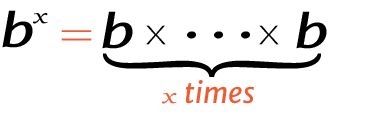# 7 raise to the power of 4

Place the base and exponent in the power calculator to get the right answer for value of 4^7, 47 (4 power 7), or 7 raised to the power of 4.

## How to calculate 4 raise to the power of 7?

This is how you can solve (4) 7 manually.

Solution:

Step 1: Simplify the data.

Base
ExponentStep 2: Multiply the 4 to itself 7 times.

Using this exponents calculator could be much easier to figure out an exponential expression.